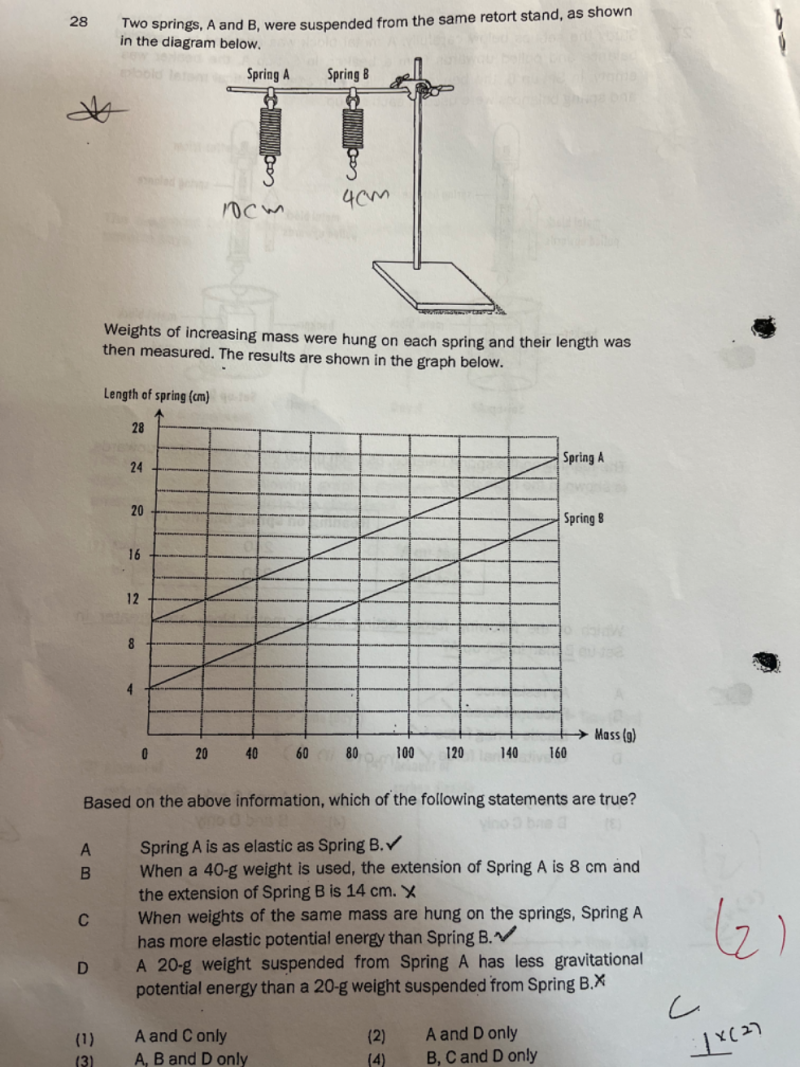# Questionhi ,can help me please? Thanks

Elastic PE is dependent on the k=spring constant(same spring) and x= the distance displaced.

Elastic PE = ½ k Δx2

Both setup showed that the spring is elastic, however, it did not state that the springs are identical so the spring constant may not be the same.  In that case, (C) may not be true because we cannot use the distance displaced only to ascertain the elastic PE.

In (D)  gravitational PE = mgh    where g is a constant anywhere on earth.

m is given as the same for both cases and hence PE varies with h.  Spring A stretches longer so the distance from spring to the ground is shorter hence its gPE is less than Spring B.   Since the potential energy is lesser, imagine a weight falling from that height, the impact would be lesser than one falling at a higher height for the same weight.

0 Replies 1 Like